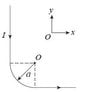# Biot-Savart Law problem

• Eva01
In summary, a long wire segment is connected to a quarter of a circular arc with a radius of 0.69 m. The other end of the arc is connected to another long horizontal wire segment. The current is flowing from the top coming down vertically and flows to the right along the positive x-axis. Using the Biot Savart law, the magnetic field at point O is calculated to be 6.8295 N/m. However, this does not take into account the additional factor required for the infinitesimal length of the arc.

## Homework Statement

A long (effectively infinite) wire segment
is connected to a quarter of a circular arc with
radius a. The other end of the arc is connected
to another long horizontal wire segment. The
current is flowing from the top coming down
vertically and flows to the right along the pos-
itive x-axis. I have included the image below.
I = 7.5A, a = 0.69 m. Find the magnetic field at O?

B = μ0 * I / 2a

## The Attempt at a Solution

As far as I understand since we only consider the arc part then I use the formula from above but I am not getting the right answer.

B = 4∏ * 10^-7 * 7.5 / 2*0.69 = 6.8295 N/m

#### Attachments

•Untitled.jpg
3.1 KB · Views: 385
Hello Eva01,

Firstly you need to consider the origin of your equation :
B = μ0 * I / 2a

which is the Biot Savart law.

$B = \frac{\mu_{0}}{4 \pi}\int \frac{(I dl \times \hat{r})}{ |\vec{r}|^{2}}$

For your system you do only need to use the arc system but you should find out why..

For the arc itself, consider the law above. Clearly the I and the denominator are constant. So we need to solve

$\int (dl \times \hat{r})$

Remembering that a chord (r) connecting the point O to the arc will always be perpendicular to an infinitesimal length of the arc. This should give you a similar equation to what you have been using but it requires an additional factor somewhere.

I would also advise that you check your units at some point.

Hope this helps